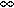# Error Comparison of Diamond Difference, Nodal, and Characteristic Methods for Solving Multidimensional Transport Problems with the Discrete Ordinates Approximation

Nuclear Science and Engineering / Volume 156 / Number 2 / June 2007 / Pages 139-153

Technical Paper / dx.doi.org/10.13182/NSE05-91

Error norms for three variants of Larsen's benchmark problem are evaluated using three numerical methods for solving the discrete ordinates approximation of the neutron transport equation in multidimensional Cartesian geometry. The three variants of Larsen's test problem are concerned with the incoming flux boundary conditions: unit incoming flux on the left and bottom edges (Larsen's configuration); unit incoming flux only on the left edge; unit incoming flux only on the bottom edge. The three methods considered are the diamond-difference (DD) method, the arbitrarily high order transport (AHOT) method of the nodal type (AHOT-N), and of the characteristic type (AHOT-C). The last two methods are employed in constant, linear, and quadratic orders of spatial approximation. The cell-wise error is computed as the difference between the cell-averaged flux computed by each method and the exact value, then the L1, L2, and Lerror norms are calculated. The new result of this study is that while integral error norms, i.e., L1 and L2, converge to zero with mesh refinement, the cellwise Lnorm does not. Via numerical experiments we relate this behavior to solution discontinuity across the singular characteristic. Little difference is observed between the error norm behavior of the methods in spite of the fact that AHOT-C is locally exact, suggesting that numerical diffusion across the singular characteristic is the major source of error on the global scale. Nevertheless, increasing the order of spatial approximation in AHOT methods yields higher accuracy in the integral error norms sense. In general, the characteristic methods possess a given accuracy in a larger fraction of the number of computational cells compared to nodal methods or DD.